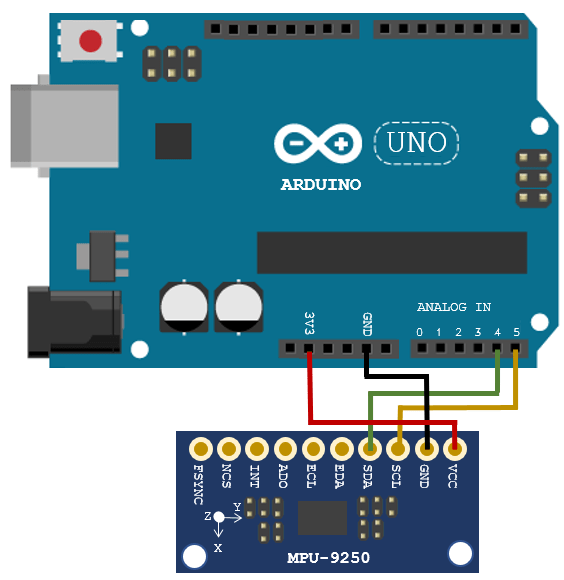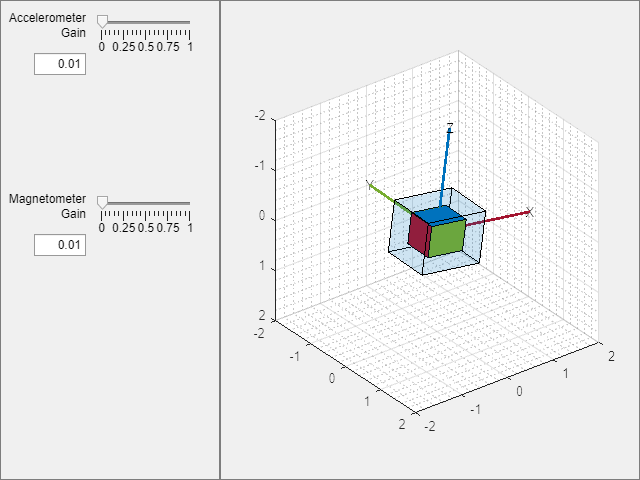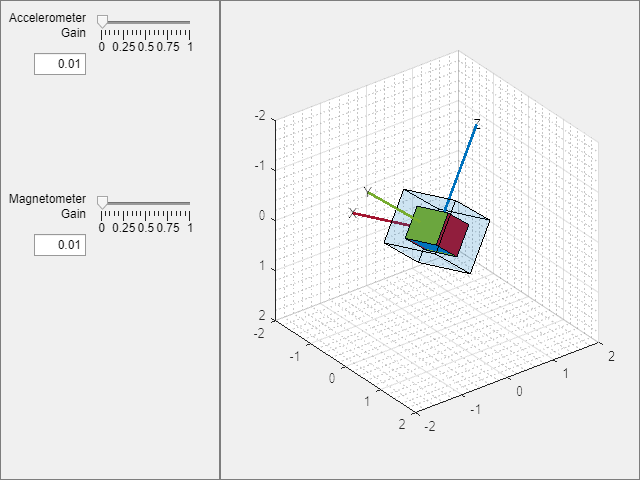# Estimate Orientation with a Complementary Filter and IMU Data

This example shows how to stream IMU data from an Arduino and estimate orientation using a complementary filter.

### Connect Hardware

Connect the SDA, SCL, GND, and VCC pins of the MPU-9250 sensor to the corresponding pins of the Arduino® hardware. This example uses an Arduino® Uno board with the following connections:

• SDA - A4

• SCL - A5

• VCC - +3.3V

• GND - GNDEnsure that the connections to the sensors are intact. It is recommended to attach/connect the sensor to a prototype shield to avoid loose connections while the sensor is in motion. Refer the Troubleshooting Sensors (MATLAB Support Package for Arduino Hardware) page to debug the sensor related issues.

### Create Sensor Object

Create an `arduino` object and an `mpu9250` object. Specify the sensor sampling rate `Fs` and the amount of time to run the loops. Optionally, enable the `isVerbose` flag to check if any samples are overrun. By disabling the `useHW` flag, you can also run the example with sensor data saved in the MAT-file `loggedMPU9250Data.mat`.

The data in `loggedMPU9250Data.mat` was logged while the IMU was generally facing due South, then rotated:

• +90 degrees around the z-axis

• -180 degrees around the z-axis

• +90 degrees around the z-axis

• +90 degrees around the y-axis

• -180 degrees around the y-axis

• +90 degrees around the y-axis

• +90 degrees around the x-axis

• -270 degrees around the x-axis

• +180 degrees around the x-axis

Notice that the last two rotations around the x-axis are an additional 90 degrees. This was done to flip the device upside-down. The final orientation of the IMU is the same as the initial orientation, due South.

```Fs = 100; samplesPerRead = 10; runTime = 20; isVerbose = false; useHW = true; if useHW a = arduino; imu = mpu9250(a, 'SampleRate', Fs, 'OutputFormat', 'matrix', ... 'SamplesPerRead', samplesPerRead); else load('loggedMPU9250Data.mat', 'allAccel', 'allGyro', 'allMag', ... 'allT', 'allOverrun', ... 'numSamplesAccelGyro', 'numSamplesAccelGyroMag') end ```

### Align Axes of MPU-9250 Sensor with NED Coordinates

The axes of the accelerometer, gyroscope, and magnetometer in the MPU-9250 are not aligned with each other. Specify the index and sign x-, y-, and z-axis of each sensor so that the sensor is aligned with the North-East-Down (NED) coordinate system when it is at rest. In this example, the magnetometer axes are changed while the accelerometer and gyroscope axes remain fixed. For your own applications, change the following parameters as necessary.

```% Accelerometer axes parameters. accelXAxisIndex = 1; accelXAxisSign = 1; accelYAxisIndex = 2; accelYAxisSign = 1; accelZAxisIndex = 3; accelZAxisSign = 1; % Gyroscope axes parameters. gyroXAxisIndex = 1; gyroXAxisSign = 1; gyroYAxisIndex = 2; gyroYAxisSign = 1; gyroZAxisIndex = 3; gyroZAxisSign = 1; % Magnetometer axes parameters. magXAxisIndex = 2; magXAxisSign = 1; magYAxisIndex = 1; magYAxisSign = 1; magZAxisIndex = 3; magZAxisSign = -1; % Helper functions used to align sensor data axes. alignAccelAxes = @(in) [accelXAxisSign, accelYAxisSign, accelZAxisSign] ... .* in(:, [accelXAxisIndex, accelYAxisIndex, accelZAxisIndex]); alignGyroAxes = @(in) [gyroXAxisSign, gyroYAxisSign, gyroZAxisSign] ... .* in(:, [gyroXAxisIndex, gyroYAxisIndex, gyroZAxisIndex]); alignMagAxes = @(in) [magXAxisSign, magYAxisSign, magZAxisSign] ... .* in(:, [magXAxisIndex, magYAxisIndex, magZAxisIndex]); ```

If necessary, you may calibrate the magnetometer to compensate for magnetic distortions. For more details, see the Compensating for Hard Iron Distortions section of the Estimating Orientation Using Inertial Sensor Fusion and MPU-9250 example.

### Specify Complementary filter Parameters

The `complementaryFilter` has two tunable parameters. The `AccelerometerGain` parameter determines how much the accelerometer measurement is trusted over the gyroscope measurement. The `MagnetometerGain` parameter determines how much the magnetometer measurement is trusted over the gyroscope measurement.

```compFilt = complementaryFilter('SampleRate', Fs) ```
```compFilt = complementaryFilter with properties: SampleRate: 100 AccelerometerGain: 0.0100 MagnetometerGain: 0.0100 HasMagnetometer: 1 OrientationFormat: 'quaternion' ```

### Estimate Orientation with Accelerometer and Gyroscope

Set the `HasMagnetometer` property to `false` to disable the magnetometer measurement input. In this mode, the filter only takes accelerometer and gyroscope measurements as inputs. Also, the filter assumes the initial orientation of the IMU is aligned with the parent navigation frame. If the IMU is not aligned with the navigation frame initially, there will be a constant offset in the orientation estimation.

```compFilt = complementaryFilter('HasMagnetometer', false); tuner = HelperOrientationFilterTuner(compFilt); if useHW tic else idx = 1:samplesPerRead; overrunIdx = 1; end while true if useHW [accel, gyro, mag, t, overrun] = imu(); accel = alignAccelAxes(accel); gyro = alignGyroAxes(gyro); else accel = allAccel(idx,:); gyro = allGyro(idx,:); mag = allMag(idx,:); t = allT(idx,:); overrun = allOverrun(overrunIdx,:); idx = idx + samplesPerRead; overrunIdx = overrunIdx + 1; pause(samplesPerRead/Fs) end if (isVerbose && overrun > 0) fprintf('%d samples overrun ...\n', overrun); end q = compFilt(accel, gyro); update(tuner, q); if useHW if toc >= runTime break; end else if idx(end) > numSamplesAccelGyro break; end end end ```### Estimate Orientation with Accelerometer, Gyroscope, and Magnetometer

With the default values of `AccelerometerGain` and `MagnetometerGain`, the filter trusts more on the gyroscope measurements in the short-term, but trusts more on the accelerometer and magnetometer measurements in the long-term. This allows the filter to be more reactive to quick orientation changes and prevents the orientation estimates from drifting over longer periods of time. For specific IMU sensors and application purposes, you may want to tune the parameters of the filter to improve the orientation estimation accuracy.

```compFilt = complementaryFilter('SampleRate', Fs); tuner = HelperOrientationFilterTuner(compFilt); if useHW tic end while true if useHW [accel, gyro, mag, t, overrun] = imu(); accel = alignAccelAxes(accel); gyro = alignGyroAxes(gyro); mag = alignMagAxes(mag); else accel = allAccel(idx,:); gyro = allGyro(idx,:); mag = allMag(idx,:); t = allT(idx,:); overrun = allOverrun(overrunIdx,:); idx = idx + samplesPerRead; overrunIdx = overrunIdx + 1; pause(samplesPerRead/Fs) end if (isVerbose && overrun > 0) fprintf('%d samples overrun ...\n', overrun); end q = compFilt(accel, gyro, mag); update(tuner, q); if useHW if toc >= runTime break; end else if idx(end) > numSamplesAccelGyroMag break; end end end ```### Summary

This example showed how to estimate the orientation of an IMU using data from an Arduino and a complementary filter. This example also showed how to configure the IMU and discussed the effects of tuning the complementary filter parameters.